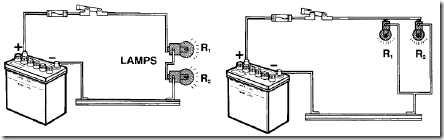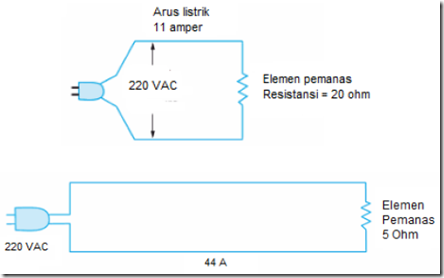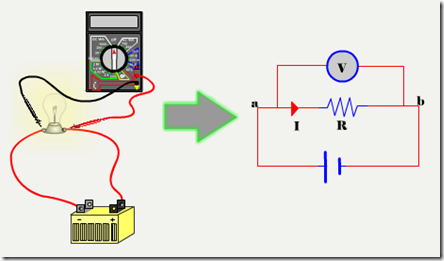Home > English > Electricity Check

# Electricity Check

Electrical equipment or electrical load in the electrical circuit that produces a particular work commonly represented as the resistance of a circuit. Figure 5.2 shows two electrical circuits that have different resistance.Figure 5.1 The lights in the Series and Parallel Relations

Any electrical equipment has a certain resistance. The amount of resistance depends on the size and equipment needs. As maintenance personnel, should know the value of resistance of each component are handled. If the value of resistannya different from a predetermined value, the electrical components may otherwise be damaged.Figure 5.2 Example of Electric Circuits

In practice, some of the industry uses to determine the unit ohm resistance values. To measure the resistance value in small scale commonly used measure m-ohms, where 1 m-ohms (mA) = 0.001 ohms (Ω). In contrast to measure the resistance in a large scale, used kiloohm size, where 1 kiloohm (kΩ) = 1000 ohm (Ω), and even to measure the strength of the insulation commonly used measure megaohm, where 1 megaohm (MΩ) = 1,000,000 ohms.

As you already know that energy is an ability to do business. Associated with electricity, to move a number of potential charges to other potential one, in which both the potential to have a different nailai, it takes energy. Consider the following picture:Figure 5.3 The series of Incandescent Lamps

5.3 Consider the picture above. On the picture looks an incandescent lamp which has a resistance R is connected to a source of electrical voltage (accumulator) giving rise to the voltage Vab between the ends of the lamp or in other words, the voltage difference between the ends of the light R becomes V with a strong current of I flows during the time interval Δt. The amount of electrical energy supplied by a voltage source for moving cargo on the lamp filament resistance R is expressed by the equation
W = V. ΔQ or W = V. I. Δt

In this case the W is the energy generated by a voltage source if the source voltage to generate electric current of I amperes in a second time interval Δt with a potential difference of V volts.
By applying Ohm’s Law in an electrical circuit (I = V / R), then the equation for the electric energy can be written in other forms such as the following
W = V. I. Δt
W = I. R. I. Δt
W = I 2. R. Δt
W = V 2 / R. Δt"Tasikmalaya, Indonesia"